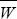# Evolution Chapter 17 Notes

NOTE 17E: In general, there is no simple relationship between the net fitness (as measured by the leading eigenvalue) and the components of a structured population model.

NOTE 17F: The variance is calculated as the average squared deviation from the mean (1/ni (Wi)2. Often, the variance is estimated as [1/(n – 1)]Σi(Wi)2, which is almost the same for large n (see Box 28.4).

NOTE 17G: The additive genetic variance can also be calculated by summing the variance contributed by the two loci and summing over the two genomes, as 2((0.65)2 + (0.1)2) = 0.867. The slight difference arises because of slight associations (i.e., linkage disequilibria) between the two loci: The red allele is slightly more likely to be found with the black allele and blue with green, which slightly reduces the variance of breeding value.

NOTE 17H: There will also be a slight discrepancy because of deviations from Hardy–Weinberg proportions and linkage equilibrium. If + alleles and – alleles tended to pair up in +– heterozygotes or in +– chromosomes, then there would be less variation in fitness and less change in allele frequency than expected from the Fundamental Theorem. We examine the consequences of such negative associations for the evolution of sex and recombination in Chapter 23.

NOTE 17I: In such cases, when each allele can increase from low frequency, we say that there is a protected polymorphism (see Chapter 18 Web Notes).

NOTE 17J: Natural selection will maximize mean fitness only if the fitness of each genotype stays constant (see p. 474). If fitnesses change with allele frequency, then mean fitness can decrease, as in this example. In other words, with frequency-dependent selection, populations do not evolve uphill on the adaptive landscape.

NOTE 17K: This formula differs from that for additive selection or for selection on haploids (Box 28.2) by the extra term (1/p0) – (1/p1). If the initial frequency is very small (p0 = 10–6, say), then this is far larger than the last logarithmic term (1/p0 = 106, but log(1/p0) = 13.8). Because a recessive allele is rarely exposed to selection when it is rare, it takes a very long time to increase from low frequency.

NOTE 17L: An exact calculation, made by integrating the differential equation as above, gives 7770 generations. This is substantially shorter, mainly because the strong advantage in the homozygote greatly shortens the time taken to go from 0.1 to 0.99. However, the rough estimate of 13,800 generations is much closer to the exact figure of 7770 than the time taken for a strictly recessive allele of ~106 generations.

NOTE 17M: This assumption of linkage equilibrium is not strictly accurate, because the mixing of populations and selection against the combination of alleles APBP (epistasis) generate linkage disequilibria. However, if there is no linkage, these are broken down to a low level by recombination.

NOTE 17N: In diploids, the same expression applies, except that it is divided by a factor of 2.

NOTE 17O: This is an approximate calculation, because it assumes that the distributions are normal and that selection is not too strong.